• Slides: 12Fundamentals of Data Representation Hexadecimal Computer Science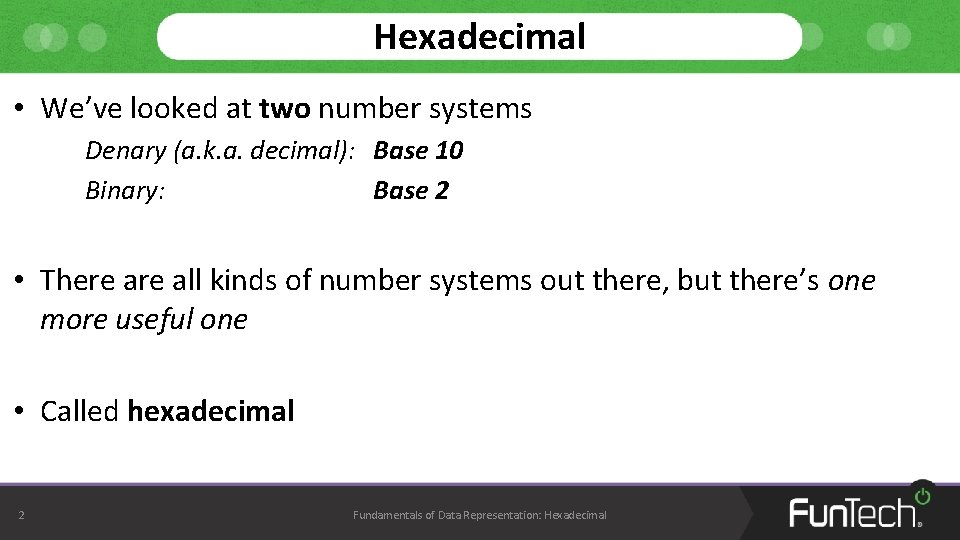Hexadecimal • We’ve looked at two number systems Denary (a. k. a. decimal): Base 10 Binary: Base 2 • There all kinds of number systems out there, but there’s one more useful one • Called hexadecimal 2 Fundamentals of Data Representation: Hexadecimal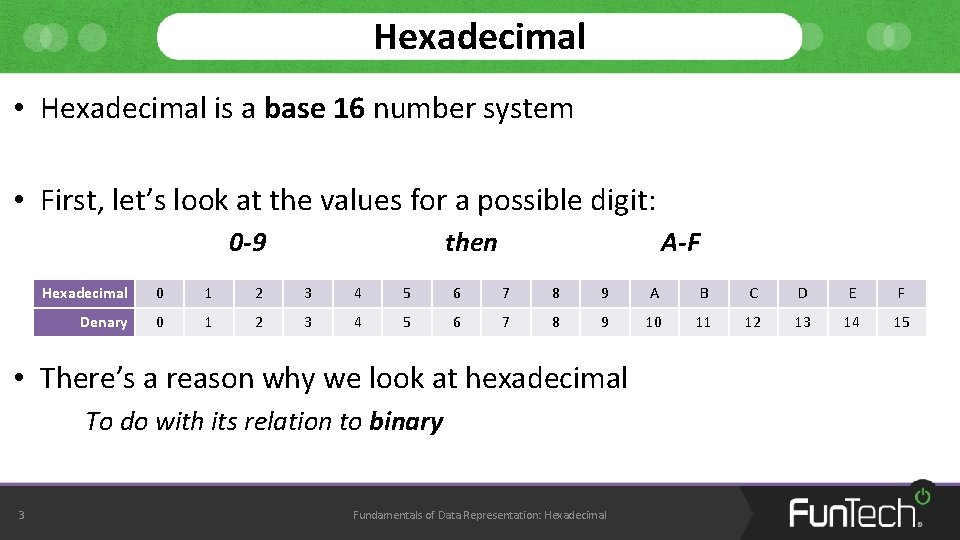Hexadecimal • Hexadecimal is a base 16 number system • First, let’s look at the values for a possible digit: 0 -9 then A-F Hexadecimal 0 1 2 3 4 5 6 7 8 9 A B C D E F Denary 0 1 2 3 4 5 6 7 8 9 10 11 12 13 14 15 • There’s a reason why we look at hexadecimal To do with its relation to binary 3 Fundamentals of Data Representation: HexadecimalHexadecimal • Let’s look at the same values – But this time we’ll include the binary values Hexadecimal 0 1 2 3 4 5 6 7 8 9 A B C D E F Denary 0 1 2 3 4 5 6 7 8 9 10 11 12 13 14 15 0001 0010 0011 0100 0101 0110 0111 1000 1001 1010 1011 1100 1101 1110 1111 Binary 0000 • Notice how the denary value 15 fits into 4 binary bits, but 1 hexadecimal digit? 4 Fundamentals of Data Representation: Hexadecimal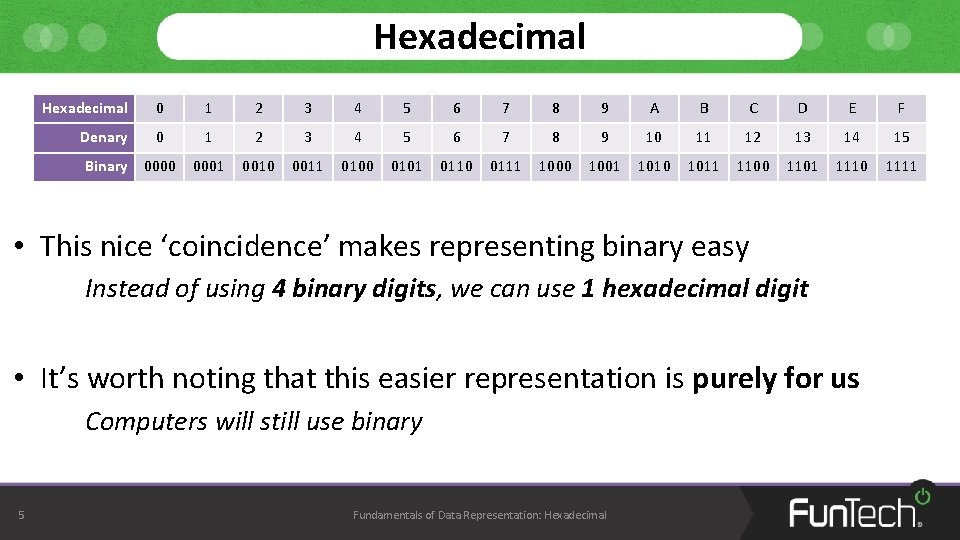Hexadecimal 0 1 2 3 4 5 6 7 8 9 A B C D E F Denary 0 1 2 3 4 5 6 7 8 9 10 11 12 13 14 15 0001 0010 0011 0100 0101 0110 0111 1000 1001 1010 1011 1100 1101 1110 1111 Binary 0000 • This nice ‘coincidence’ makes representing binary easy Instead of using 4 binary digits, we can use 1 hexadecimal digit • It’s worth noting that this easier representation is purely for us Computers will still use binary 5 Fundamentals of Data Representation: HexadecimalConverting to Hexadecimal • As we have another number system, we’ll need to know how to convert From denary to hexadecimal From binary to hexadecimal From hexadecimal to denary From hexadecimal to binary • Luckily, the best way to convert between hexadecimal and denary is to go through binary So we only need to work out how to go between hexadecimal and binary Denary 6 Binary Fundamentals of Data Representation: Hexadecimal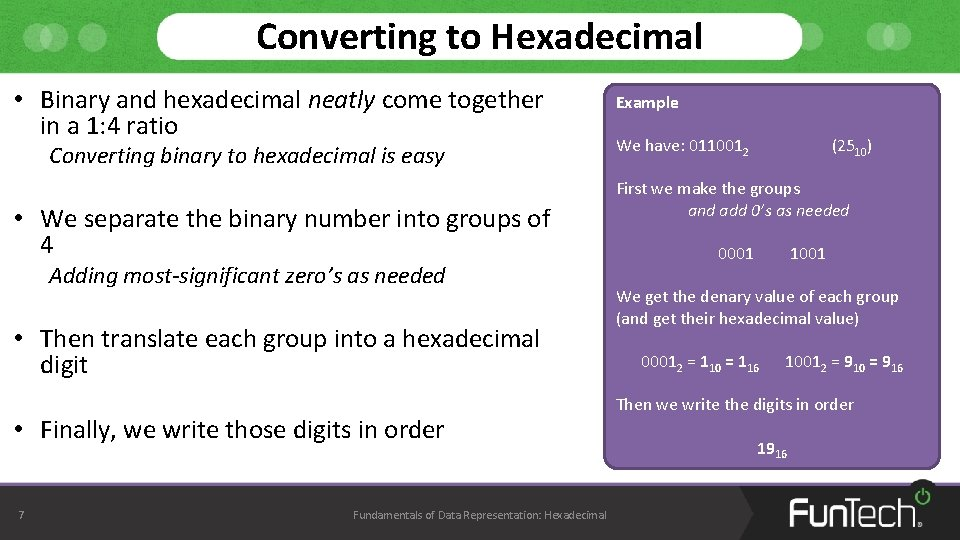Converting to Hexadecimal • Binary and hexadecimal neatly come together in a 1: 4 ratio Converting binary to hexadecimal is easy • We separate the binary number into groups of 4 Adding most-significant zero’s as needed • Then translate each group into a hexadecimal digit • Finally, we write those digits in order 7 Fundamentals of Data Representation: Hexadecimal Example We have: 0110012 (2510) First we make the groups and add 0’s as needed 0001 1001 We get the denary value of each group (and get their hexadecimal value) 00012 = 110 = 116 10012 = 910 = 916 Then we write the digits in order 1916• Convert the following denary values into hexadecimal 8 28 11 39 150 200 255 Fundamentals of Data Representation: HexadecimalConverting from Hexadecimal • Converting from hexadecimal to binary works in the exact opposite way Example • We split the hexadecimal number into its digits First we split the number into its digits (and convert each one into denary) • We find the denary value of each digit • We convert that denary value into a 4 -bit binary number • Finally, we write the binary numbers in order From left-to-right 9 Fundamentals of Data Representation: Hexadecimal We have: A 516 A 16 = 1010 516 = 510 Then we convert each number into 4 -bit binary 1010 = 10102 510 = 01012 Then we write these numbers on after the other 101001012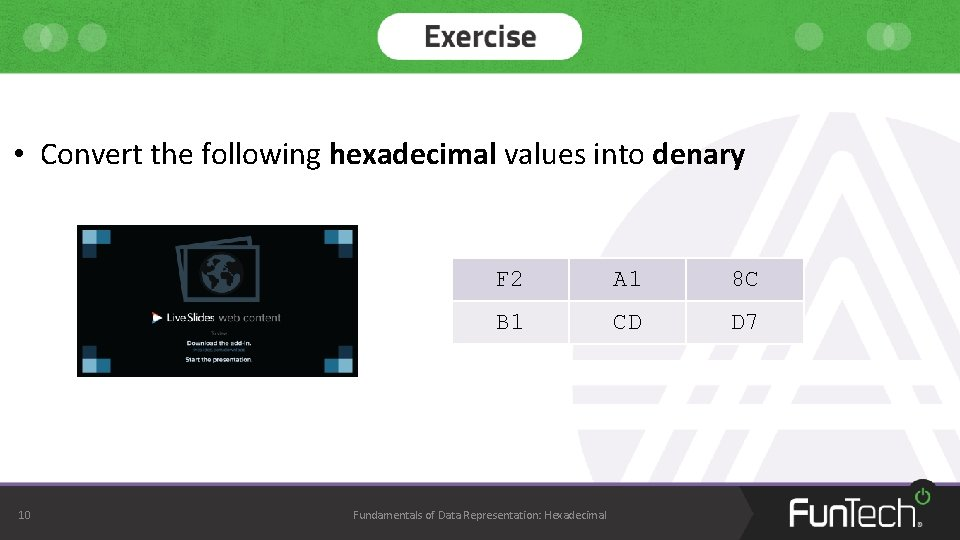• Convert the following hexadecimal values into denary 10 F 2 A 1 8 C B 1 CD D 7 Fundamentals of Data Representation: Hexadecimal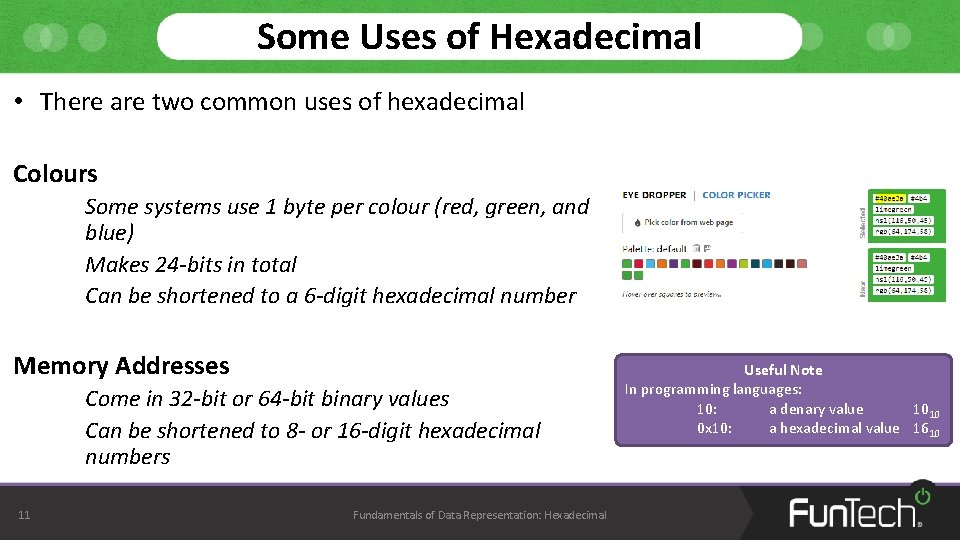Some Uses of Hexadecimal • There are two common uses of hexadecimal Colours Some systems use 1 byte per colour (red, green, and blue) Makes 24 -bits in total Can be shortened to a 6 -digit hexadecimal number Memory Addresses Come in 32 -bit or 64 -bit binary values Can be shortened to 8 - or 16 -digit hexadecimal numbers 11 Fundamentals of Data Representation: Hexadecimal Useful Note In programming languages: 10: a denary value 1010 0 x 10: a hexadecimal value 1610